# Basic Right Triangle Trigonometry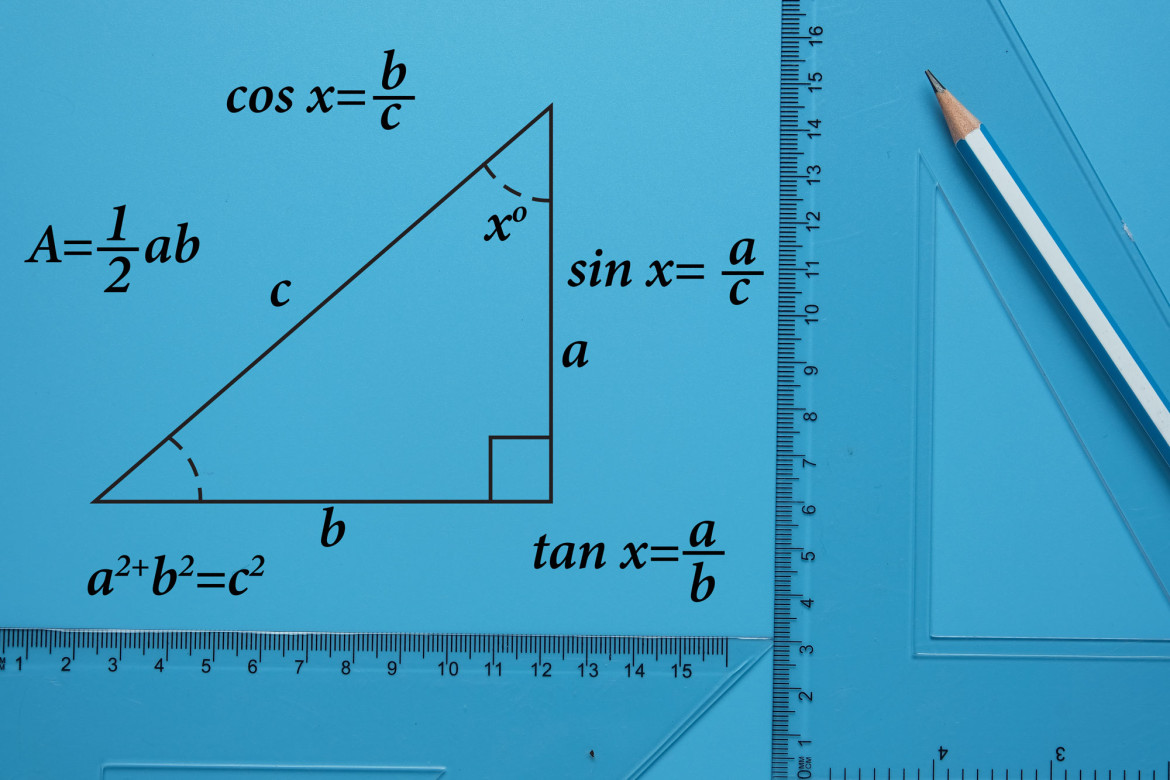This is a brief review of the basic trig functions Sine, Cosine, and Tangent as applied to a right triangle. A right triangle is a triangle where one angle measures 90°.

In order to calculate the Sine, Cosine and Tangent of an angle, first identify one angle.  We will indicate this angle with the Greek letter “theta” or: Θ.

## Label the sides of the triangle:

1. The side opposite the right angle is the hypotenuse.
2. The side opposite the angle Θ, is called the “opposite” side.
3. The remaining side is called the “adjacent” side.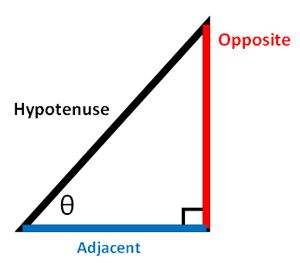The sine of the angle, SIN Θ, is the ratio of the opposite side of the triangle to the hypotenuse of the triangle.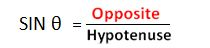The cosine of the angle, COS Θ, is the ratio of the adjacent side of the triangle divided by the hypotenuse of the triangle.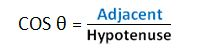The tangent of the angle, TAN Θ, is the ratio of the opposite side of the triangle divided by the adjacent side of the triangle.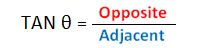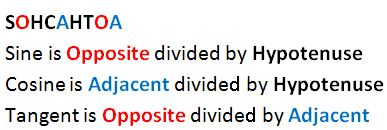These ratios are the same for any similar triangles, or any triangles with the same angle measurements.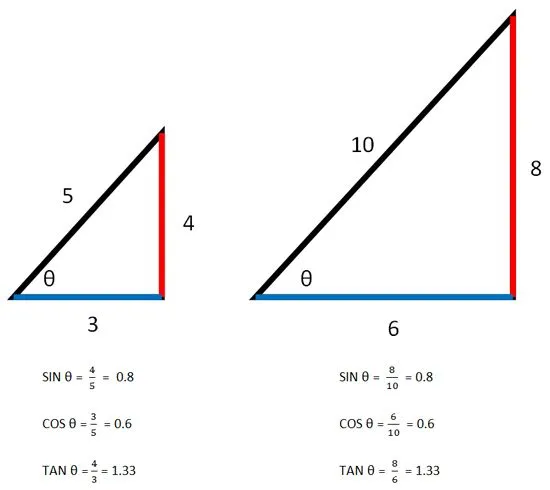Note:  The sine, cosine, and tangent of an angle have no units. If the measurements of the triangles above were in meters, then the units would cancel out.